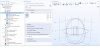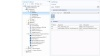COMSOL® 中声固耦合的建模

2019年 6月 12日

可用于声固耦合分析的物理场接口• 压力声学
• 压力波在黏性边界层之外的流体域中并且处于静止的条件下
• 热黏性声学
• 在声学的详细建模过程中，要包括壁附近的边界层，其中黏性损失和热传导不可忽略
• 气动声学
• 在静止环境下的声学改变就意味着流动，也称为对流声学
• 超声
• 使用不连续的Galerkin方法可以在比波长长度更大的距离里传播声信号
• 几何声学
• 高频声学，其声学波长远小于其几何特征使用预定义的多物理场接口对声固耦合进行建模ASI的耦合方法

-\textbf
{n} \cdot \Big(-\frac{1}{\rho_c}(\nabla p_t – q_d)\Big) = -\textbf{n}
\cdot \textbf
{u}_{tt}
\textbf{F}_A = p_t\textbf{n}

-\textbf{n} \cdot \Big(-\frac{1}{\rho_c}(\nabla p_t – q_d)\Big)_{up} = -\textbf{n} \cdot \textbf{u}
_
{tt}
-\textbf{n} \cdot \Big(-\frac{1}{\rho_c}(\nabla p_t – q_d)\Big)_{down} = -\textbf{n} \cdot \textbf{u}_{tt}
\textbf
{F}
_A = (p_t,down – p_t,up)\textbf
{n}

• 声学结构边界
• 热黏性声学结构边界
• 气动声学结构边界
• 声-孔边界
• 多孔结构边界

通过手动添加物理接口和耦合来对ASI进行建模示例：对集总接收器进行建模intop_in()在细管的入口表面上定义了一个积分算子，其中，p 是声压，acpr.iomega 是复合角频率，和 cir.V2_i是 SPICE 接收器输出处的电流。解决大型ASI模型下一步

• 在 COMSOL 博客上了解有关声学建模的更多信息：